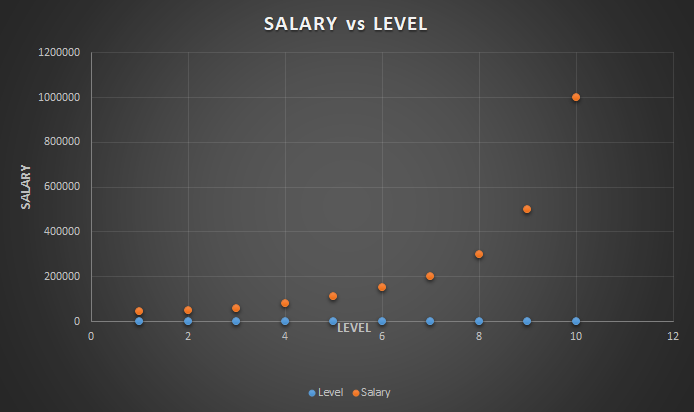Polynomial Regression | Machine Learning

Polynomial Regression: Polynomial regression is a form of regression analysis in which the relationship between the independent variable x and dependent variable y is modelled as an nth degree polynomial of x. That is, if your dataset holds the characteristic of being curved when plotted in the graph, then you should go with a polynomial regression model instead of Simple Linear or Multiple Linear regression.

The equation for Polynomial Regression looks very similar to that of Multiple Linear Regression.

y=b0+b1*x1+b2*(x1)2+....+bn*(x1)n

The main difference is that in Multiple Linear Regression, there are several variables with the same degree but here the single variable has different powers.

Why Polynomial Regression?

Lets say you have a dataset and fit both Simple and Polynomial Regression to that data.Plotted with a linear regression model

Here you can see the data has a tendency to grow in a non-linear fashion. Hence a simple linear model could not find the most optimal line that can fit the data well and has a very poor accuracy level.Plotted with a polynomial regression model

Now if you look at the Polynomial Regression, you will clearly see the difference. The Polynomial model has fitted the dataset well with a higher accuracy rate than that of a simple linear model.

There are many cases where you will find great uses of Polynomial Regression. For example, if you want to discover how diseases spread, how a pandemic or epidemic spread over a continent and so on. It completely depends on your data. And based on the non-linear characteristics of your data, you should use  Polynomial Regression.

Now we will jump into a dataset and implement this idea.

Suppose we have a dataset(Salary_data.csv) which contains the salaries of employees of different positions based on their Level. Lets have a look at the dataset.You can download the dataset from here.

If we plot the above data in a graph, it would look like this-Here, you can observe that our data has a tendency of growing non linearly. So we are required to use a Polynomial Regression for this case.

The dataset contains just three columns. We will take the second column, Level as our independent variable in feature matrix, X and the Salary column as the dependent variable in the dependent variable vector, y.

We start by preprocessing the data. For this use this following codes-

# Polynomial Regression
# Importing the Essential Libraries
import numpy as np
import matplotlib.pyplot as plt import pandas as pd
# Importing the dataset
X = dataset.iloc[:, 1].values
y = dataset.iloc[:, 2].values

We skip the splitting part as the dataset contains only 10 values. So lets fit polynomial regression to our whole dataset and for this you should write the following code-

# Fitting Polynomial Regression to the dataset
from sklearn.preprocessing import PolynomialFeatures
poly_reg = PolynomialFeatures(degree = 4)
X_poly = poly_reg.fit_transform(X)
poly_reg.fit(X_poly, y)
lin_reg_2 = LinearRegression()
lin_reg_2.fit(X_poly, y)

Note: Here, I use degree = 4, you should try with different values for this parameter and watch how your model works. For this dataset, a value of degree 4 just works fine!

Well, we have fitted our model to the dataset. Now, its time to plot and see how it works.

# Visualising the Polynomial Regression results
X_grid = np.arange(min(X), max(X), 0.1)
X_grid = X_grid.reshape((len(X_grid), 1))
plt.scatter(X, y, color = 'red')
plt.plot(X_grid, lin_reg_2.predict(poly_reg.fit_transform(X_grid)), color = 'blue')
plt.title('Truth or Bluff (Polynomial Regression)')
plt.xlabel('Position level')
plt.ylabel('Salary')
plt.show()

The plot should look like this-With a 4-degree polynomial regression, we obtained a model that has closely predicted the Salary values. If we used a simple linear regression instead, we could not obtain this level of accuracy.

So now we can use our model to learn how it predicts for an unknown value.

# Predicting an unknown value with Polynomial Regression model
y_pred = lin_reg_2.predict(poly_reg.fit_transform([[6.5]]))

For a level value of 6.5, it predicts: 158862

We put the same dataset in a simple linear regression model. And for the same level value of 6.5, it gave us the outcome: 330378.78!

If you compare both the value with the first SALARY vs LEVEL graph, you must discover that our polynomial model has predicted a way better than that of a simple linear regression model.